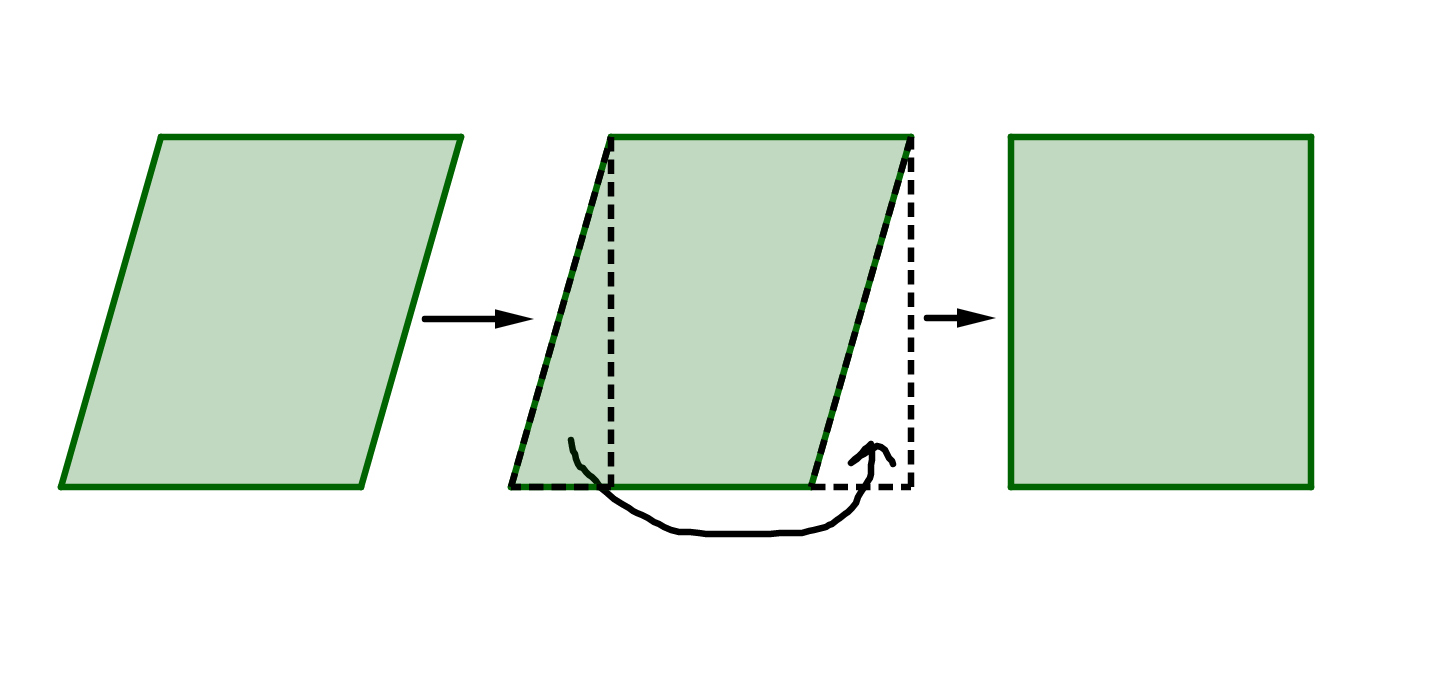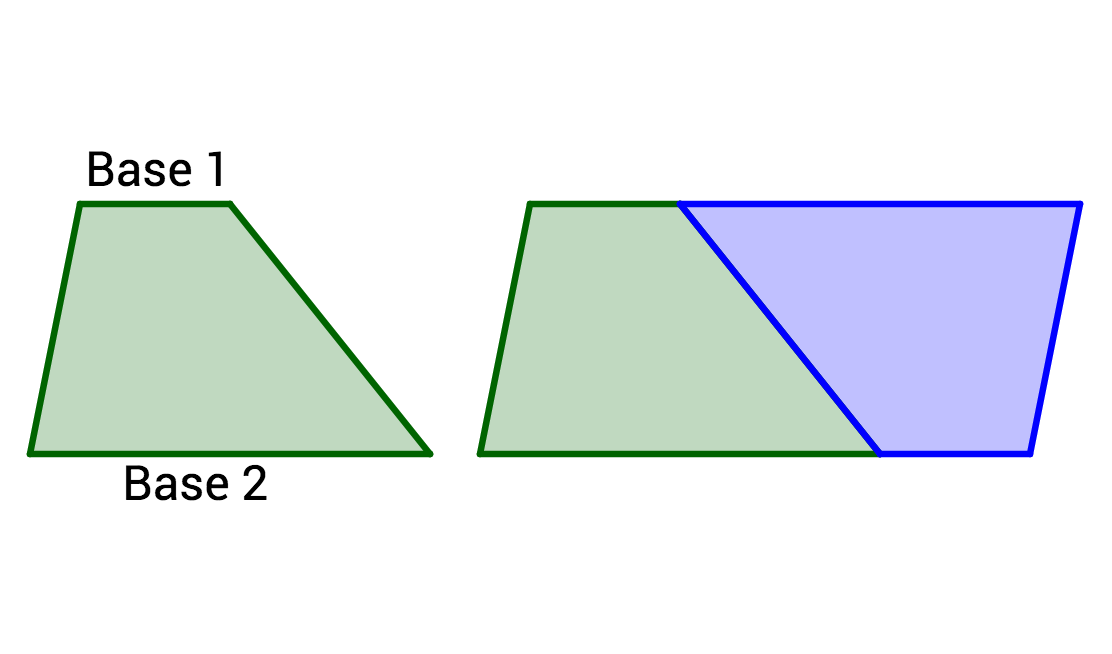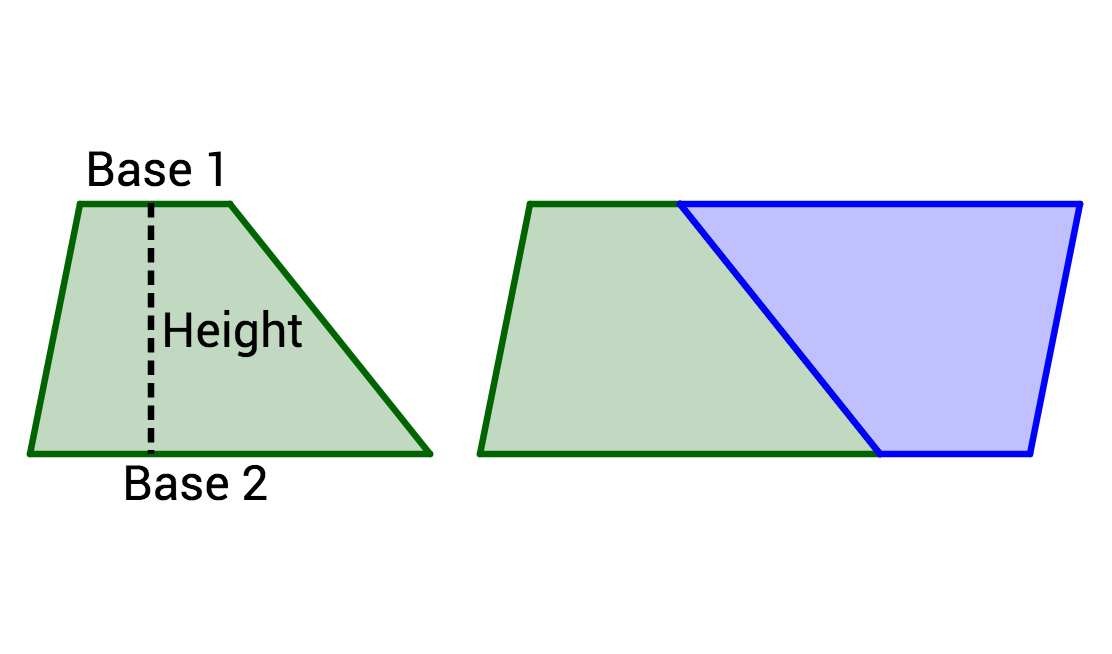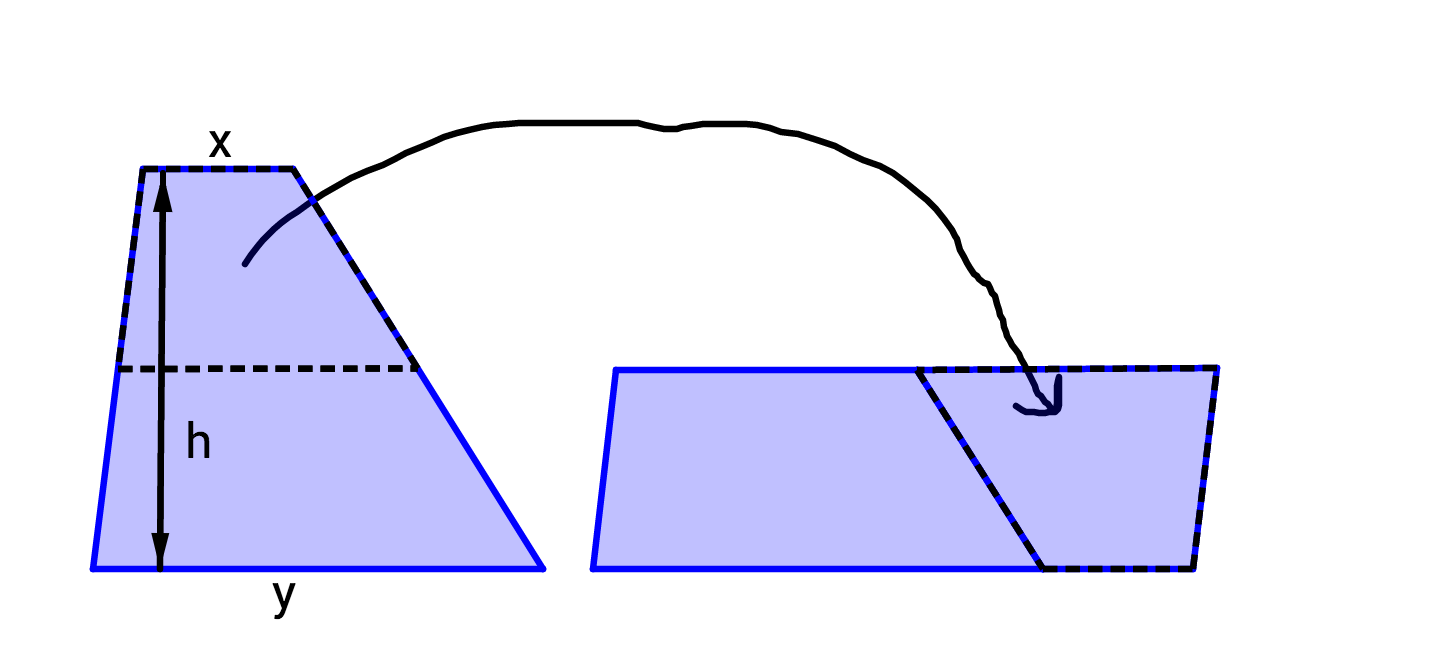Geometry

# Area From Rectangles to Parallelograms and TrapezoidsIn the parallelogram above, we drew a perpendicular line from one vertex to the opposite side, then cut that piece off of the parallelogram, and added it to the other side of the parallelogram to create a rectangle. Can the pieces of a parallelogram always be combined like this to create a rectangle?A parallelogram is converted to a rectangle by cutting and rearranging pieces of the shape, as shown above. Given that the area of a rectangle is $$\text{(base)}\times\text{(height)},$$ is the area of a parallelogram also $$\text{(base)}\times\text{(height)}?$$The parallelogram was created by duplicating the trapezoid, rotating it, and adding it on to itself. What is the base length of the parallelogram?Two identical trapezoids combined to form the parallelogram, so the area of the trapezoid is half of the area of the parallelogram. Given the base lengths of Base 1 and Base 2, what is the area of the trapezoid?A trapezoid can be cut into two pieces and arranged into a parallelogram as shown. The two figures have the same area. Does this expression represent the area? $\frac{1}{2}(h)(x + y)$

×

Problem Loading...

Note Loading...

Set Loading...